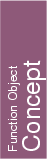## Kernel::Equal_3

A model for this must provide the following operations. For all of them fo(x,y) returns true iff x and y are equal.

bool
 fo.operator() ( Kernel::Point_3 x, Kernel::Point_3 y)

bool
 fo.operator() ( Kernel::Vector_3 x, Kernel::Vector_3 y)

bool
 fo.operator() ( Kernel::Direction_3 x, Kernel::Direction_3 y)

bool fo.operator() ( Kernel::Line_3 x, Kernel::Line_3 y)

bool
 fo.operator() ( Kernel::Plane_3 x, Kernel::Plane_3 y)

bool fo.operator() ( Kernel::Ray_3 x, Kernel::Ray_3 y)

bool
 fo.operator() ( Kernel::Segment_3 x, Kernel::Segment_3 y)

bool
 fo.operator() ( Kernel::Sphere_3 x, Kernel::Sphere_3 y)

bool
 fo.operator() ( Kernel::Triangle_3 x, Kernel::Triangle_3 y)

bool
 fo.operator() ( Kernel::Tetrahedron_3 x, Kernel::Tetrahedron_3 y)

bool
 fo.operator() ( Kernel::Iso_cuboid_3 x, Kernel::Iso_cuboid_3 y)

### Refines

AdaptableFunctor (with two arguments)